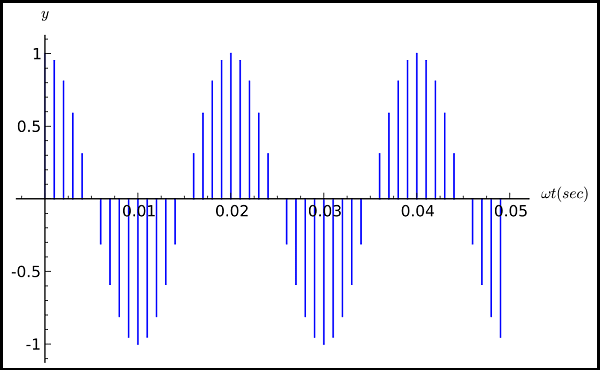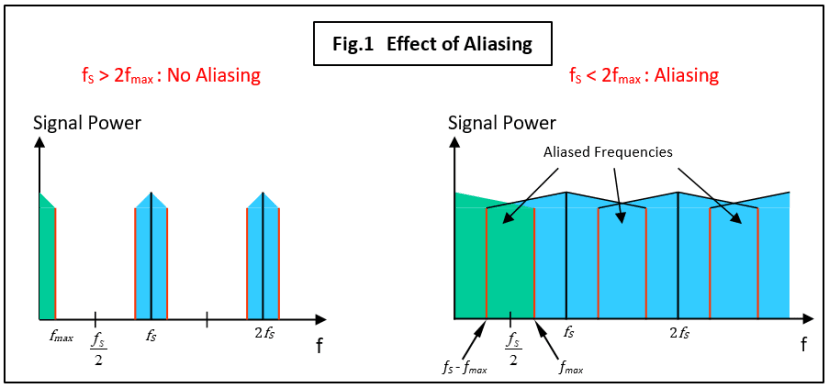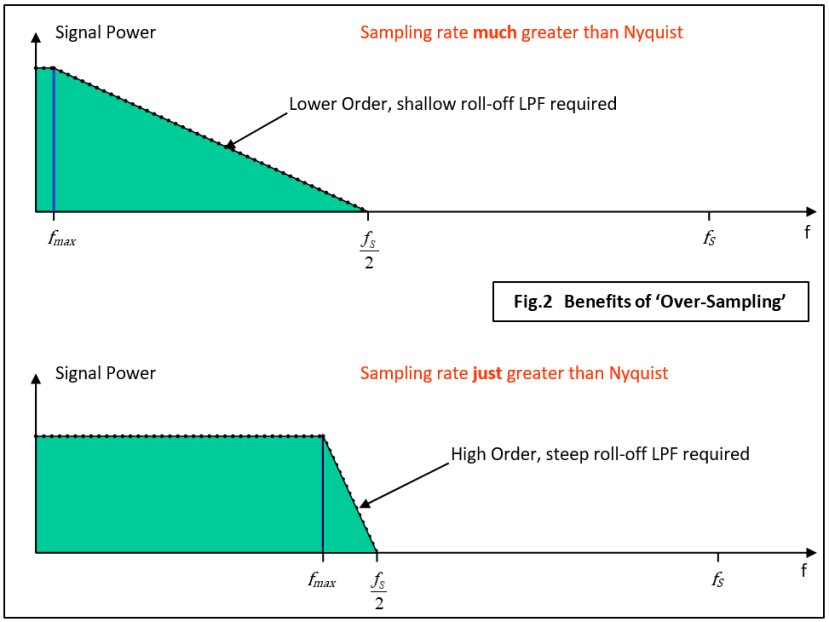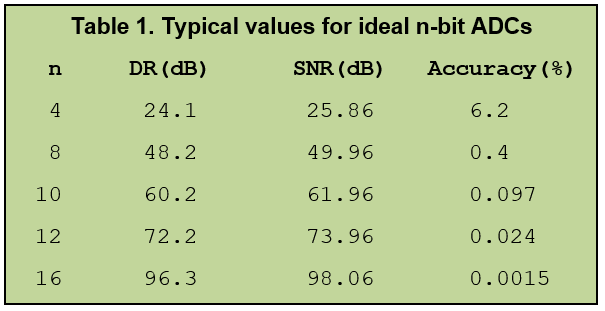# Getting into Digital Signal Processing Part 2: Sampling & AliasingIn the first part of this series, Getting into Digital Signal Processing: A Basic Introduction, I talked about why we might choose digital over analogue signal processing. Now let’s start on the how beginning with the process of selecting a suitable Analogue-Digital Converter (ADC).50Hz cosine waveform, sampled at 1000Hz                 Image credit: Wikipedia

### Get the Specification

Before an ADC is selected, the designer must have available a certain amount of numerical data:

• The maximum frequency component of the analogue signal.
• The Dynamic Range of the analogue signal (ratio of the maximum to the minimum input signal level).
• The required Signal-to-Noise ratio of the digitized signal.

### Decide on the sampling rate

The sampling rate is set according to the Nyquist criterion which states that it must be more than twice that of the maximum frequency component present in the analogue signal. This ensures accurate reproduction, but a much higher rate will ease the design of a vital circuit that precedes the ADC: the Anti-Aliasing filter.

### Design considerations for the Anti-Aliasing Filter

The frequency plots in Fig.1 provide a graphical representation of aliasing. In this example, we are going to sample a baseband signal which has a maximum frequency component of fmax (the band in green) using a sampling frequency of fS. The plot on the left shows all the new frequencies present in the non-aliased sampled signal. Note that we now have new bands of frequencies (in blue) each with a width of 2 x fmax and centred on the sampling frequency fS and its harmonics. This is a correctly sampled signal because fS > 2 x fmax. By contrast the plot on the right shows extensive aliasing where the various bands overlap leading to the production of erroneous frequencies in the green baseband.Don’t worry about all that seemingly redundant information created by the sampling process: the copies of the baseband wrapped around the sampling frequency and its harmonics. So long as no overlap occurs (aliasing), the original analogue baseband signal can be reconstructed exactly. The heading picture is a graphical representation of a simple cosine wave sampled at a high rate. Each of those vertical lines represents the sampled value at that instant - the value converted to a digital number by the ADC and stored in the computer memory. As a cosine wave has only one frequency component, the resulting frequency plot after sampling looks like Fig.1, but with only the black and red lines present. Note that, thanks to the 'zero-width' sampling pulse, the harmonics of the sampling frequency extend to infinity. In theory.

The practical problem is that few ‘raw’ signals have a nice, clean fmax. In order to avoid aliased components being produced, a low-pass anti-aliasing filter needs to be placed in the circuit before the ADC. Fig.2 shows the trade-off between the sampling rate and the order (roll-off) of the low-pass filter. The designer can massively over-sample and then use a simple low-order filter or select a lower rate and then be faced with the need for a complex multi-pole type.However, the ability of the DSP device to process the algorithm between consecutive samples must be considered before the sampling rate is set. It can save a lot of trouble later if the DSP program is tested and timed on a suitable development system before the sampling rate is fixed and the filter designed.

### A practical Anti-Aliasing Filter

A common choice of analogue filter is the Sallen-Key type (Fig.3). This circuit is for a 2-pole Butterworth filter (maximally flat in the passband) with a roll-off of 12dB/octave. It is likely that a 4 or 6-pole type will be required to achieve the necessary roll-off and fortunately, the Sallen-Key circuit can be cascaded.

If R1 = R2 = R and C1 = C2 = C then the cut-off frequency is 1/2πRC Hz. Useful gain can be had too, given by K = 1 + (R3/R4).

### ADC resolution (Number of bits/sample)

What Dynamic Range is required? Dynamic Range (DR) is the ratio of the smallest to the largest signal amplitude that will be resolved. In other words:

• DR = Vmax / Vmin = 2n or in decibels: DR = 6.02n dB where n = no. of ADC bits

You can now work out the Signal-to-Quantization Noise Ratio (SNR) associated with a particular number of bits.

• For an ideal ADC: SNR = DR +1.76 dB
• For worst-case conditions with ½ LSB of ADC linearity error: SNR = DR – 4.24 dB

Note that this SNR figure assumes that the analogue input is noiseless.Table 1. provides a quick reference guide for some common ADC sizes. It’s very tempting to go for the higher resolutions, but you can see from the table that an 8-bit ADC yields a sampling accuracy of better than 0.5%. This is adequate for most applications. Bear in mind that if the analogue signal being sampled is noisy (usually the case), then the lower significant bits of the sample become useless. So, a 16-bit ADC can theoretically resolve a very tiny signal, but if say, the 4 LSBs are swamped by noise, then the result is no better than that obtained from a 12-bit ADC.

### Real ADCs and Conversion Time

The process of analogue to digital conversion can be achieved by various methods and a glance at a few datasheets will reveal terms such as ‘Flash’ and ‘Successive Approximation’. These normally relate to the time it takes for a sample to be converted. The theoretical calculations above assume that conversion is ideal, i.e. instantaneous with tCONV = 0. Naturally, practical components take a finite time to convert during which the input may change and this becomes yet another factor in the design. It can cause serious inaccuracy if the sampling rate is close to the Nyquist limit and tCONV is a significant proportion of the sampling period. You may need a device called a Sample & Hold to achieve the required performance. Fortunately, modern microcontrollers/DSPs usually have that functionality built-in.

### Next Time in Part 3

I’ll consider the other end of our DSP system, where processed digital data is usually, but not always converted back into analogue samples by a Digital to Analogue Convertor or DAC. Any intervening digital signal processing will have just changed the shape of the baseband frequency plot: all the sampling harmonic copies will remain. Without careful design, the DAC could introduce unwanted distortion as it returns the sampled signal back to a continuous analogue form.

If you're stuck for something to do, follow my posts on Twitter. I link to interesting articles on new electronics and related technologies, retweeting posts I spot about robots, space exploration and other issues.

Engineer, PhD, lecturer, freelance technical writer, blogger & tweeter interested in robots, AI, planetary explorers and all things electronic. STEM ambassador. Designed, built and programmed my first microcomputer in 1976. Still learning, still building, still coding today.